In :
import networkx as nx
import matplotlib.pyplot as plt
from collections import Counter
from custom import load_data as cf
from custom import ecdf
import warnings
warnings.filterwarnings('ignore')
from nxviz import CircosPlot
import numpy as np

%matplotlib inline
%config InlineBackend.figure_format = 'retina'


We will load the sociopatterns network data for this notebook. From the Konect website:

This network describes the face-to-face behavior of people during the exhibition INFECTIOUS: STAY AWAY in 2009 at the Science Gallery in Dublin. Nodes represent exhibition visitors; edges represent face-to-face contacts that were active for at least 20 seconds. Multiple edges between two nodes are possible and denote multiple contacts. The network contains the data from the day with the most interactions.

In :
# Load the sociopatterns network data.

In :
# How many nodes and edges are present?
len(G.nodes()), len(G.edges())

Out:
(410, 2765)

# Hubs: How do we evaluate the importance of some individuals in a network?¶

Within a social network, there will be certain individuals which perform certain important functions. For example, there may be hyper-connected individuals who are connected to many, many more people. They would be of use in the spreading of information. Alternatively, if this were a disease contact network, identifying them would be useful in stopping the spread of diseases. How would one identify these people?

## Approach 1: Neighbors¶

One way we could compute this is to find out the number of people an individual is conencted to. NetworkX let's us do this by giving us a G.neighbors(node) function.

In :
# Let's find out the number of neighbors that individual #7 has.
len(list(G.neighbors(9)))

Out:
14

API Note: As of NetworkX 2.0, G.neighbors(node) now returns a dict_keyiterator, which means we have to cast them as a list first in order to compute its length.

### Exercise¶

Can you create a ranked list of the importance of each individual, based on the number of neighbors they have? (3 min.)

Hint: One suggested output would be a list of tuples, where the first element in each tuple is the node ID (an integer number), and the second element is the number of neighbors that it has.

Hint: Python's sorted(iterable, key=lambda x:...., reverse=True) function may be of help here.

In :
# Possible Answers:
sorted(G.nodes(),
key=lambda x:len(list(G.neighbors(x))), reverse=True)[0:5]
# sorted([(n, G.neighbors(n)) for n in G.nodes()],
#        key=lambda x: len(x), reverse=True)[0:5]

Out:
[51, 272, 235, 195, 161]

## Approach 2: Degree Centrality¶

The number of other nodes that one node is connected to is a measure of its centrality. NetworkX implements a degree centrality, which is defined as the number of neighbors that a node has normalized to the number of individuals it could be connected to in the entire graph. This is accessed by using nx.degree_centrality(G)

In :
# nx.degree_centrality(G)

# Uncomment the next line to show a truncated version.
list(nx.degree_centrality(G).items())[0:5]

Out:
[(100, 0.07090464547677261),
(101, 0.03178484107579462),
(102, 0.039119804400977995),
(103, 0.06356968215158924),
(104, 0.04156479217603912)]

If you inspect the dictionary closely, you will find that node 51 is the one that has the highest degree centrality, just as we had measured by counting the number of neighbors.

There are other measures of centrality, namely betweenness centrality, flow centrality and load centrality. You can take a look at their definitions on the NetworkX API docs and their cited references. You can also define your own measures if those don't fit your needs, but that is an advanced topic that won't be dealt with here.

The NetworkX API docs that document the centrality measures are here: http://networkx.readthedocs.io/en/networkx-1.11/reference/algorithms.centrality.html?highlight=centrality#module-networkx.algorithms.centrality

### Exercises¶

The following exercises are designed to get you familiar with the concept of "distribution of metrics" on a graph.

1. Can you create an ECDF of the distribution of degree centralities?
2. Can you create an ECDF of the distribution of number of neighbors?
3. Can you create a scatterplot of the degree centralities against number of neighbors?
4. If I have n nodes, then how many possible edges are there in total, assuming self-edges are allowed? What if self-edges are not allowed?

Exercise Time: 8 minutes.

Here is what an ECDF is (https://en.wikipedia.org/wiki/Empirical_distribution_function).

Hint: You may want to use:

ecdf(list_of_values)



to get the empirical CDF x- and y-values for plotting, and

plt.scatter(x_values, y_values)



Hint: You can access the dictionary .keys() and .values() and cast them as a list.

If you know the Matplotlib API, feel free to get fancy :).

In :
# Possible Answers:
fig = plt.figure(0)
# Get a list of degree centrality scores for all of the
# nodes in the graph
degree_centralities = list(
nx.degree_centrality(G).values())
x, y = ecdf(degree_centralities)
# Plot the histogram of degree centralities.
plt.scatter(x, y)
# Set the plot title.
plt.title('Degree Centralities')

Out:
Text(0.5,1,'Degree Centralities')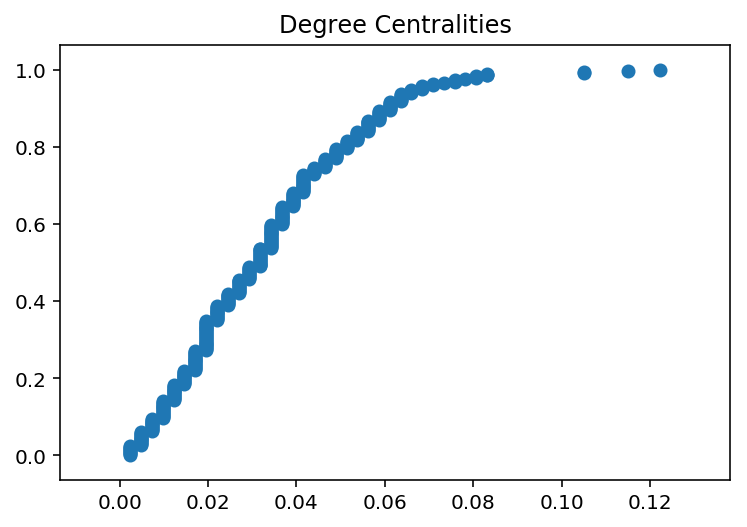In :
fig = plt.figure(1)
neighbors = [len(list(G.neighbors(node))) for node in G.nodes()]
x, y = ecdf(neighbors)
plt.scatter(x, y)
plt.title('Number of Neighbors')

Out:
Text(0.5,1,'Number of Neighbors')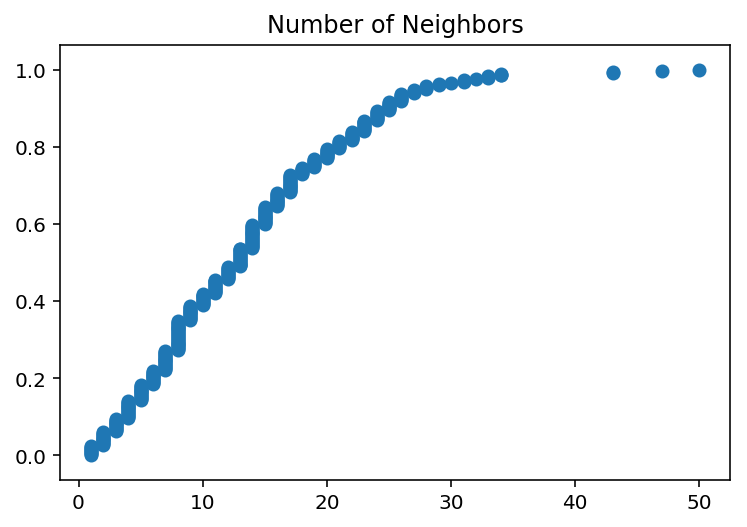In :
fig = plt.figure(2)
plt.scatter(degree_centralities, neighbors, alpha=0.1)
plt.xlabel('Degree Centralities')
plt.ylabel('Number of Neighbors')

Out:
Text(0,0.5,'Number of Neighbors')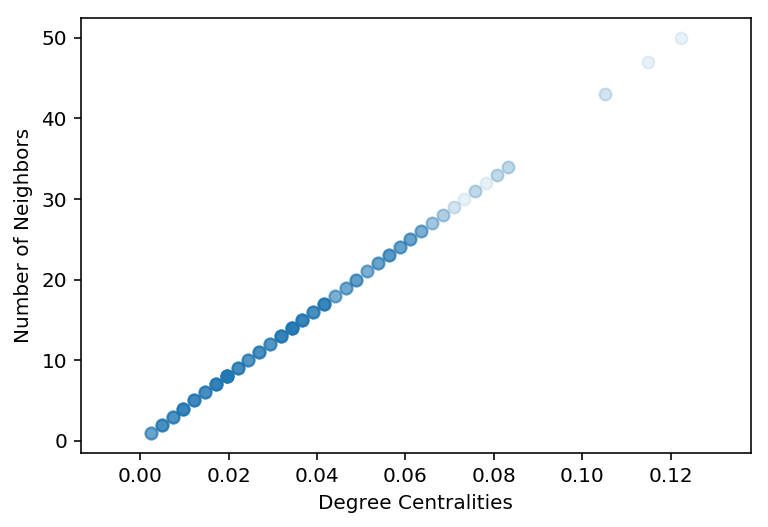### Exercise¶

Before we move on to paths in a network, see if you can use the Circos plot to visualize the network. Order and color the nodes according to the order keyword. (2 min.)

The CircosPlot API needs documentation written; for now, I am providing the following "on-the-spot" docs for you.

To instantiate and draw a CircosPlot:

c = CircosPlot(G, node_order='node_key', node_color='node_key')
c.draw()
plt.show()  # or plt.savefig(...)


Notes:

• 'node_key' is a key in the node metadata dictionary that the CircosPlot constructor uses for determining the colour, grouping, and ordering of the nodes.
• In the following exercise, you may want to use order, which is already encoded on each node in the graph.
In :
c = CircosPlot(G, node_order='order', node_color='order')
c.draw()
plt.savefig('images/sociopatterns.png', dpi=300)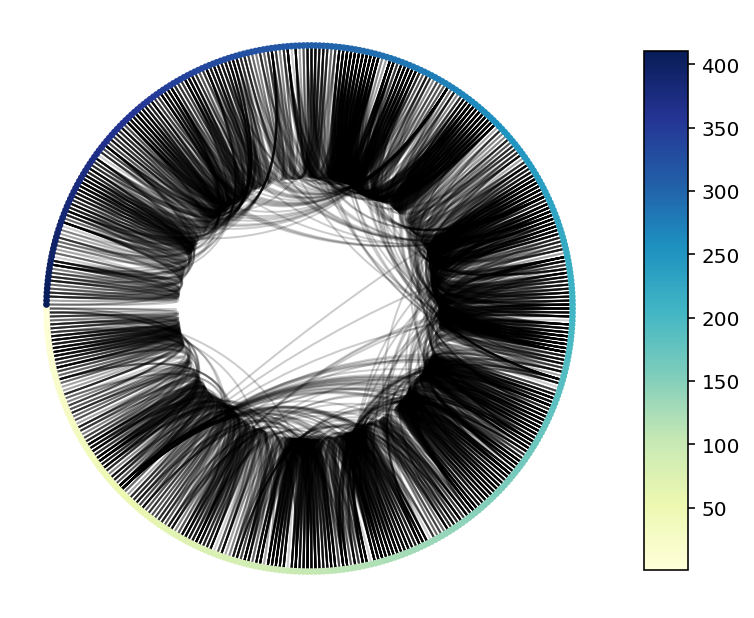What can you deduce about the structure of the network, based on this visualization?

Nodes are sorted by ID. Nodes are more connected to proximal rather than distal nodes. The data are based on people streaming through an enclosed space, so it makes sense that people are mostly connected to others proximal in order, but occasionally some oddballs stick around.

# Paths in a Network¶

Graph traversal is akin to walking along the graph, node by node, restricted by the edges that connect the nodes. Graph traversal is particularly useful for understanding the local structure (e.g. connectivity, retrieving the exact relationships) of certain portions of the graph and for finding paths that connect two nodes in the network.

Using the synthetic social network, we will figure out how to answer the following questions:

1. How long will it take for a message to spread through this group of friends? (making some assumptions, of course)
2. How do we find the shortest path to get from individual A to individual B?

## Shortest Path¶

Let's say we wanted to find the shortest path between two nodes. How would we approach this? One approach is what one would call a breadth-first search (http://en.wikipedia.org/wiki/Breadth-first_search). While not necessarily the fastest, it is the easiest to conceptualize.

The approach is essentially as such:

1. Begin with a queue of the starting node.
2. Add the neighbors of that node to the queue.
1. If destination node is present in the queue, end.
2. If destination node is not present, proceed.
3. For each node in the queue:
1. Remove node from the queue.
2. Add neighbors of the node to the queue. Check if destination node is present or not.
3. If destination node is present, end.
4. If destination node is not present, continue.

### Exercise¶

Try implementing this algorithm in a function called path_exists(node1, node2, G). (15 min.)

The function should take in two nodes, node1 and node2, and the graph G that they belong to, and return a Boolean that indicates whether a path exists between those two nodes or not. For convenience, also print out whether a path exists or not between the two nodes.

In :
def path_exists(node1, node2, G):
"""
This function checks whether a path exists between two nodes (node1,
node2) in graph G.

Special thanks to @ghirlekar for suggesting that we keep track of the
"visited nodes" to prevent infinite loops from happening. This also
removes the need to remove nodes from queue.

With thanks to @joshporter1 for the second bug fix. Originally there was
an extraneous "if" statement that guaranteed that the "False" case would
never be returned - because queue never changes in shape. Discovered at
PyCon 2017.

With thanks to @chendaniely for pointing out the extraneous "break".
"""
visited_nodes = set()
queue = [node1]

for node in queue:
if node2 in G.neighbors(node):
print('Path exists between nodes {0} and {1}'.format(node1, node2))
return True
else:
queue.extend([n for n in neighbors if n not in visited_nodes])

print('Path does not exist between nodes {0} and {1}'.format(node1, node2))
return False


In :
# Test your answer below
def test_path_exists():
assert path_exists(18, 10, G)
assert path_exists(22, 51, G)

test_path_exists()

Path exists between nodes 18 and 10
Path exists between nodes 22 and 51


If you write an algorithm that runs breadth-first, the recursion pattern is likely to follow what we have done above. If you do a depth-first search (i.e. DFS), the recursion pattern is likely to look a bit different. Take it as a challenge exercise to figure out how a DFS looks like.

Meanwhile... thankfully, NetworkX has a function for us to use, titled has_path, so we don't have to implement this on our own. :-) Check it out here.

In :
nx.has_path(G, 400, 1)

Out:
True

NetworkX also has other shortest path algorithms implemented.

We can build upon these to build our own graph query functions. Let's see if we can trace the shortest path from one node to another.

nx.shortest_path(G, source, target) gives us a list of nodes that exist within one of the shortest paths between the two nodes. (Not all paths are guaranteed to be found.)

In :
nx.shortest_path(G, 4, 400)

Out:
[4, 90, 111, 112, 132, 230, 335, 400]

Incidentally, the node list is in order as well.

### Exercise¶

Write a function that extracts the edges in the shortest path between two nodes and puts them into a new graph, and draws it to the screen. It should also return an error if there is no path between the two nodes. (5 min.)

Hint: You may want to use G.subgraph(iterable_of_nodes) to extract just the nodes and edges of interest from the graph G. You might want to use the following lines of code somewhere:

newG = G.subgraph(nodes_of_interest)
nx.draw(newG)



newG will be comprised of the nodes of interest and the edges that connect them.

In :
# Possible Answer:

def extract_path_edges(G, source, target):
# Check to make sure that a path does exists between source and target.
if nx.has_path(G, source, target):
nodes = nx.shortest_path(G, source, target)
newG = G.subgraph(nodes)
return newG

else:
raise Exception('Path does not exist between nodes {0} and {1}.'.format(source, target))

newG = extract_path_edges(G, 4, 400)
nx.draw(newG, with_labels=True)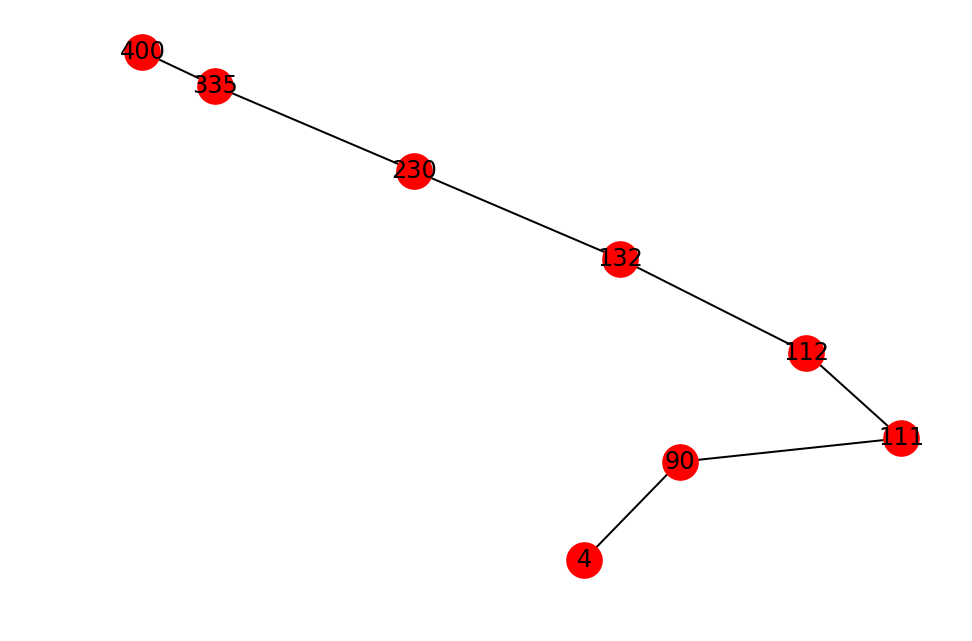### Challenge Exercise (at home)¶

These exercises below are designed to let you become more familiar with manipulating and visualizing subsets of a graph's nodes.

Write a function that extracts only node, its neighbors, and the edges between that node and its neighbors as a new graph. Then, draw the new graph to screen.

In :
# Possible Answer

def extract_neighbor_edges(G, node):
neighbors = list(G.neighbors(node))
newG = nx.Graph()

for n1, n2 in G.edges():
if (n1 == node and n2 in neighbors) or (n1 in neighbors and n2 == node):

return newG

fig = plt.figure(0)
newG = extract_neighbor_edges(G, 23)
nx.draw(newG, with_labels=True)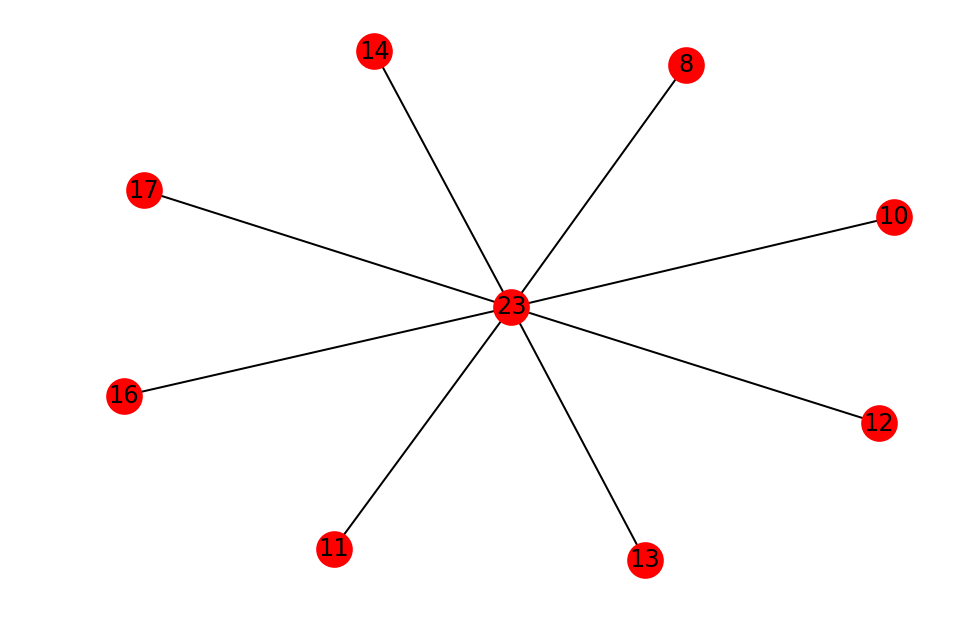In :
def extract_neighbor_edges2(G, node):
neighbors = G.neighbors(node)
newG = nx.Graph()

for neighbor in neighbors:
if (node, neighbor) in G.edges() or (neighbor, node) in G.edges():